ICSE Class 10 Maths Sample Question Paper – Mock 6 with Answers are provided with detailed explanations, along with the required images and graphs at BYJU’S. Students can download the pdf of these solutions as well as sample papers for class 10 maths for their practice as a part of their board examination preparation. These papers will help them in practising several questions similar to the board exam questions, so that they can answer them correctly in the examination.

Students can refer to the mock sample question papers of Maths along with their solutions to get acquainted with the concepts as well as solution methods, which are important from the examination point of view. Revision and practice, these are the two important things which help the students in cracking difficult exams like Maths in ICSE.

#### ICSE Class 10 Maths Mock Sample Paper 6 with Solutions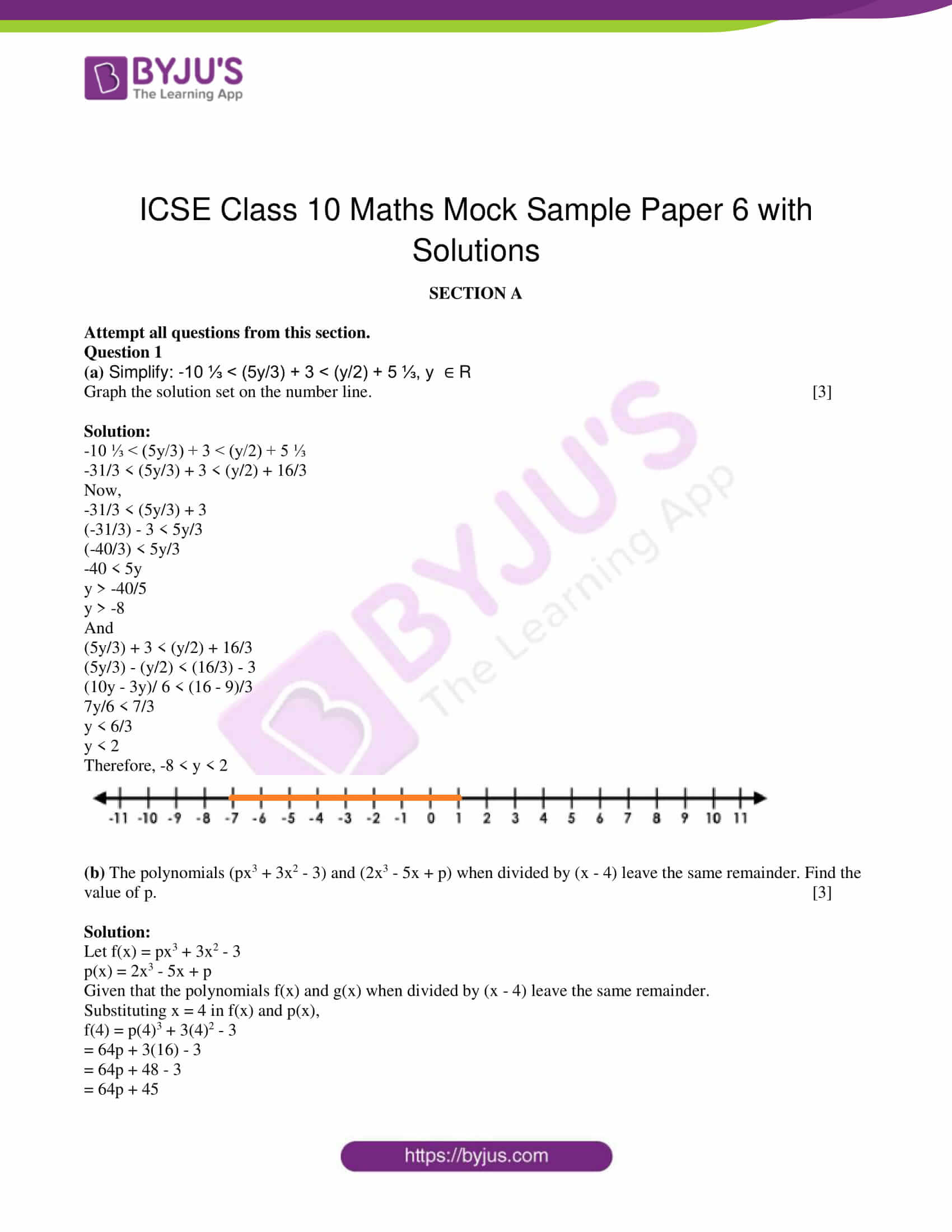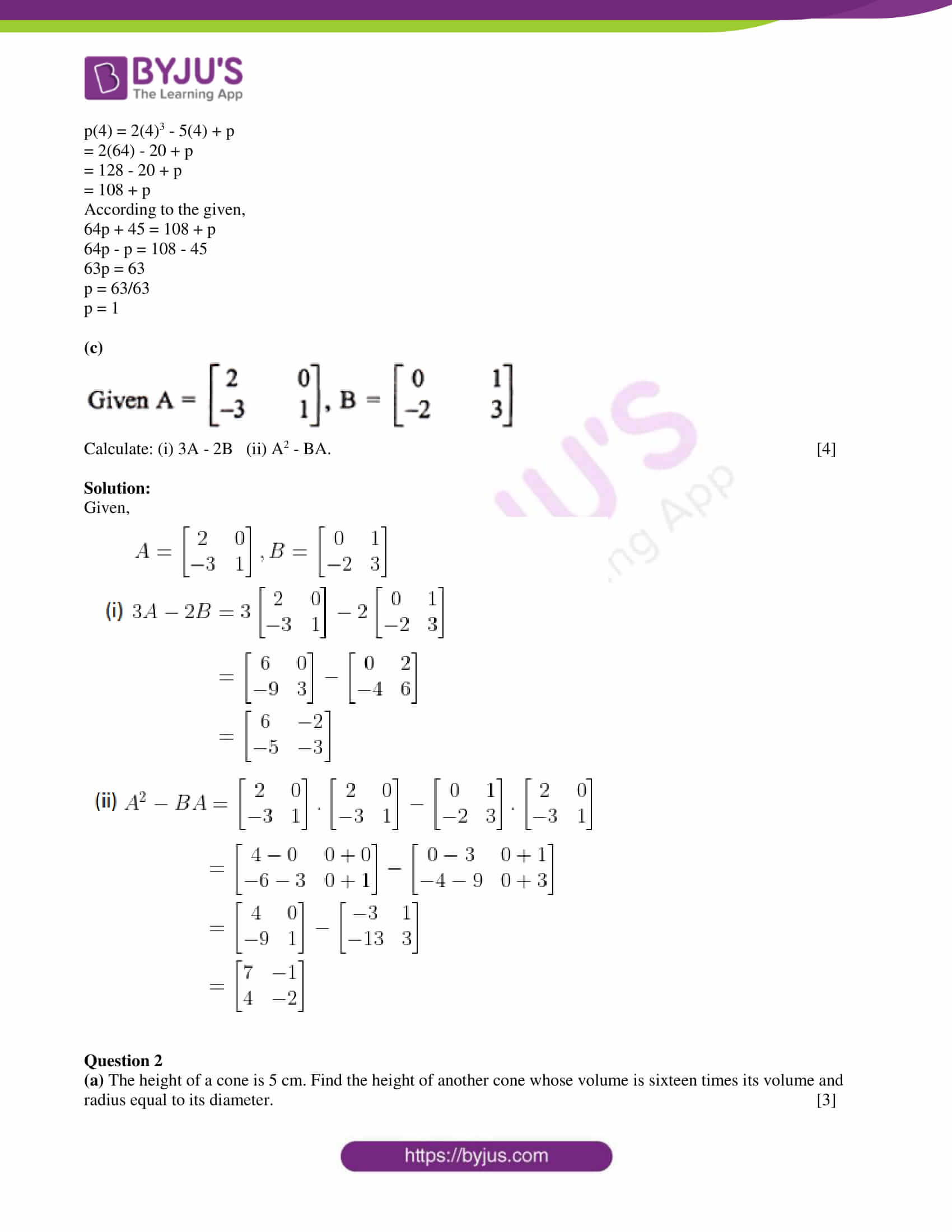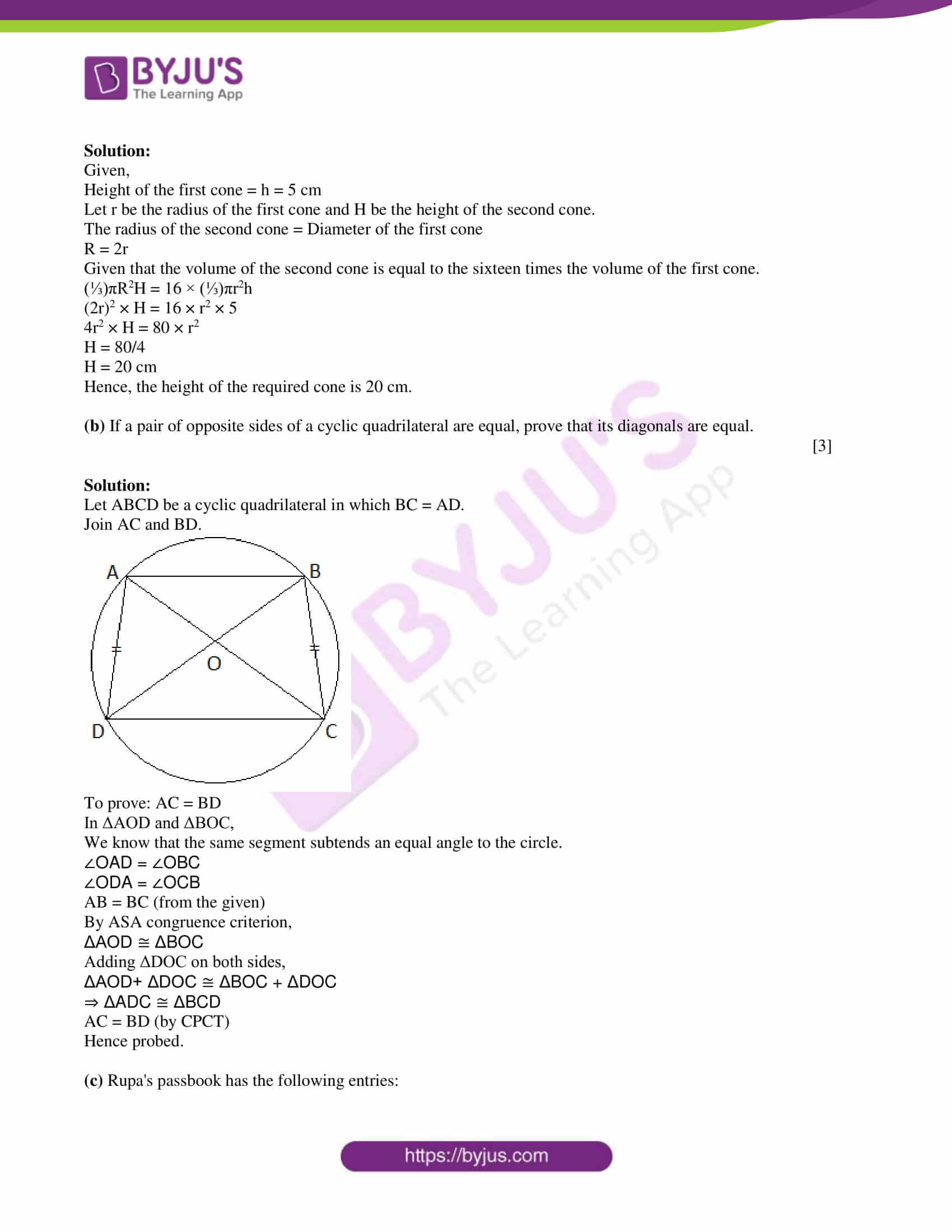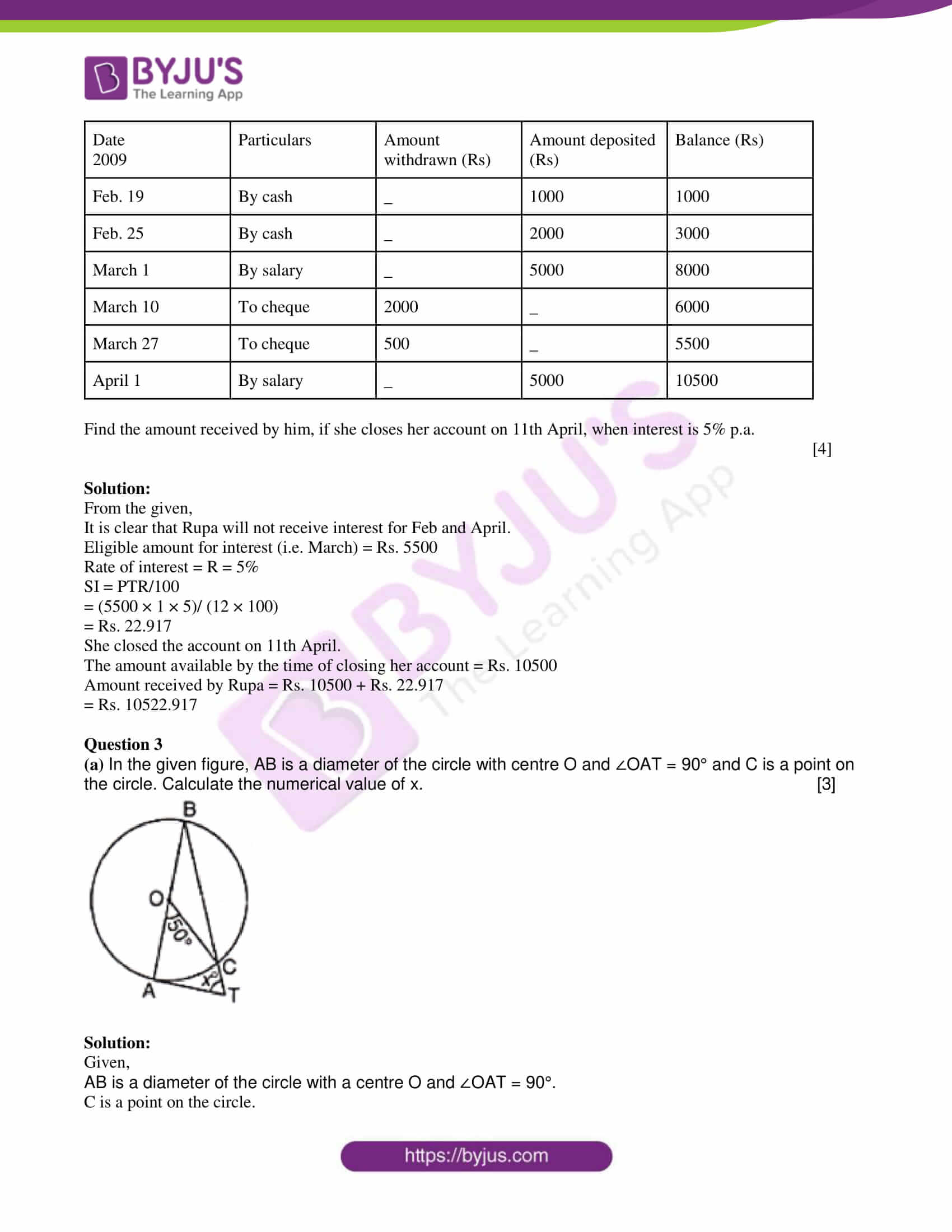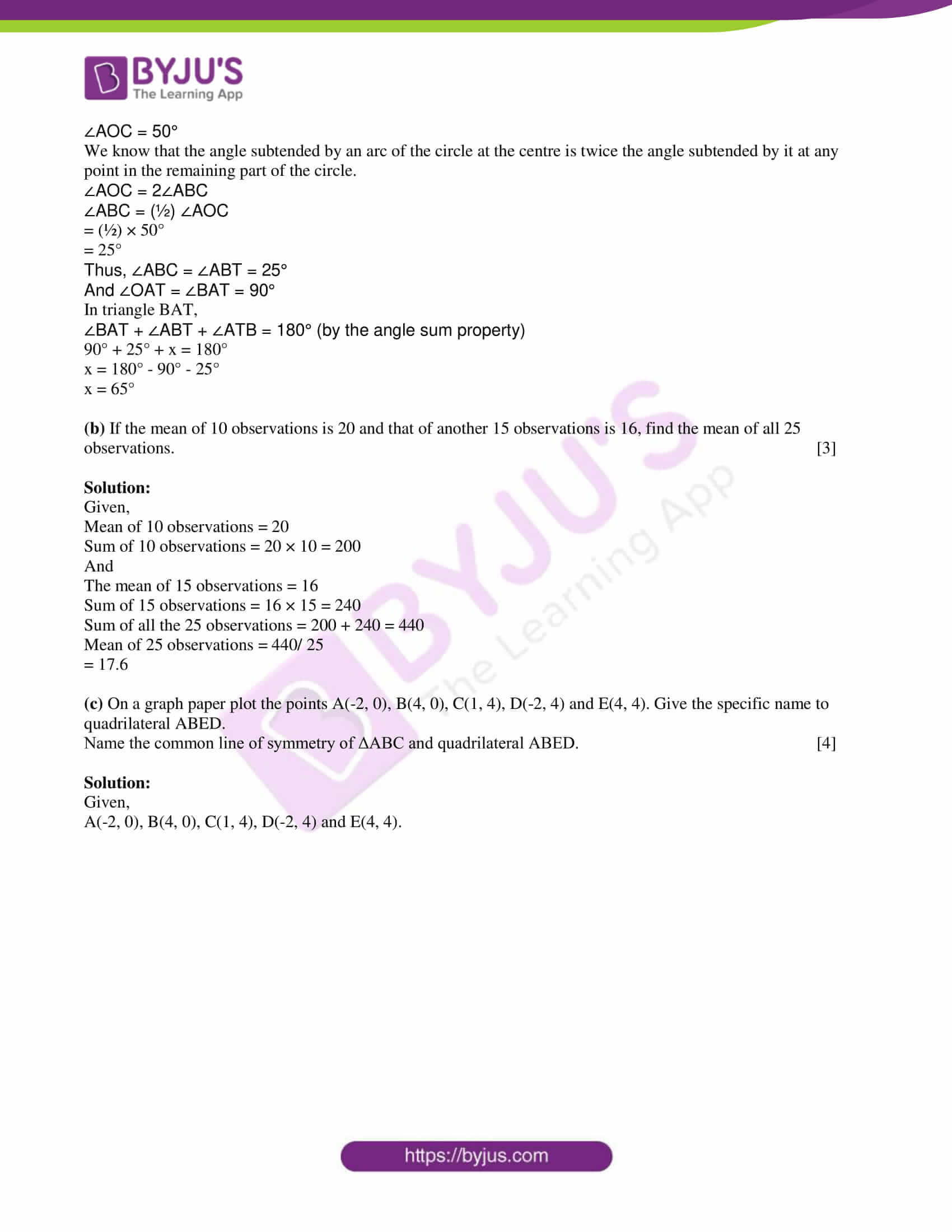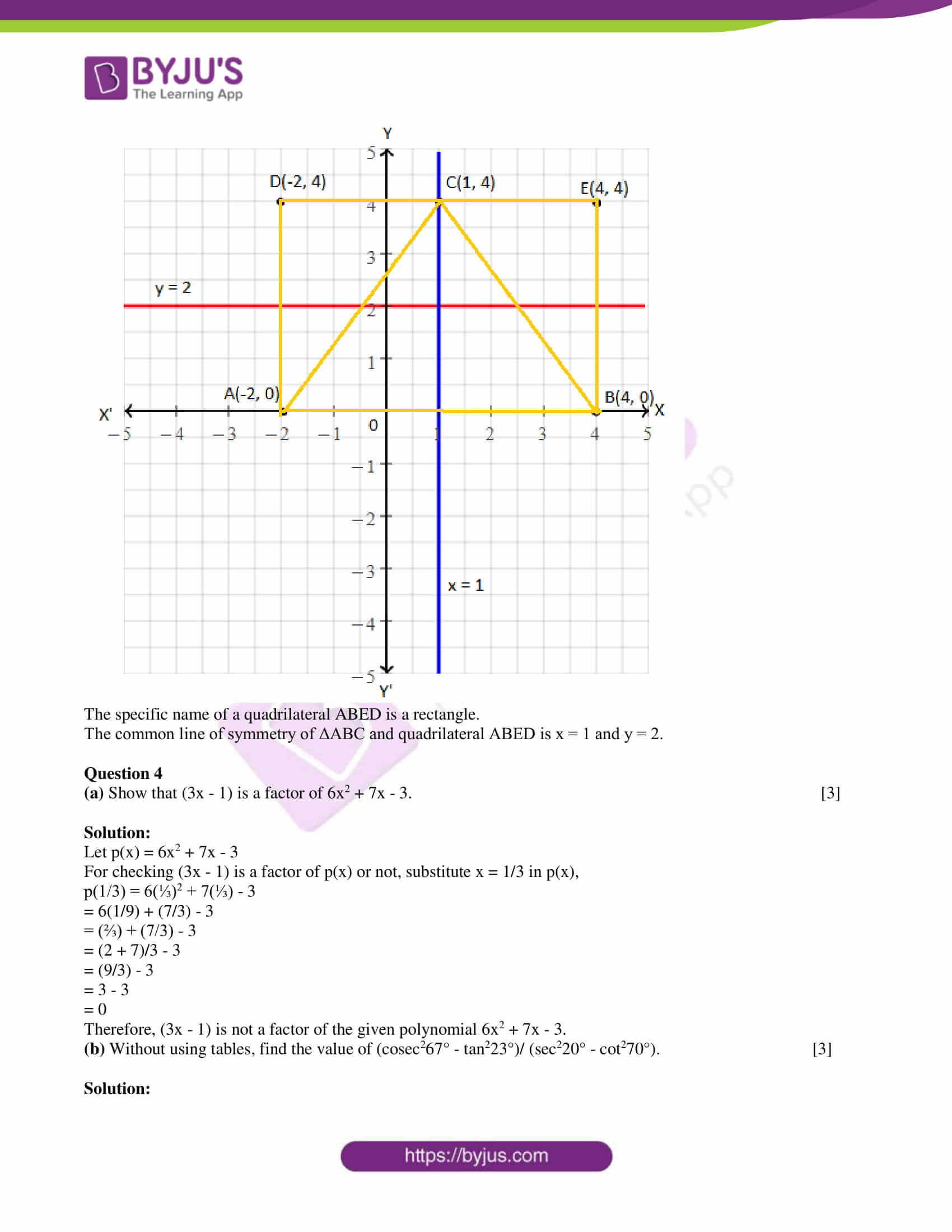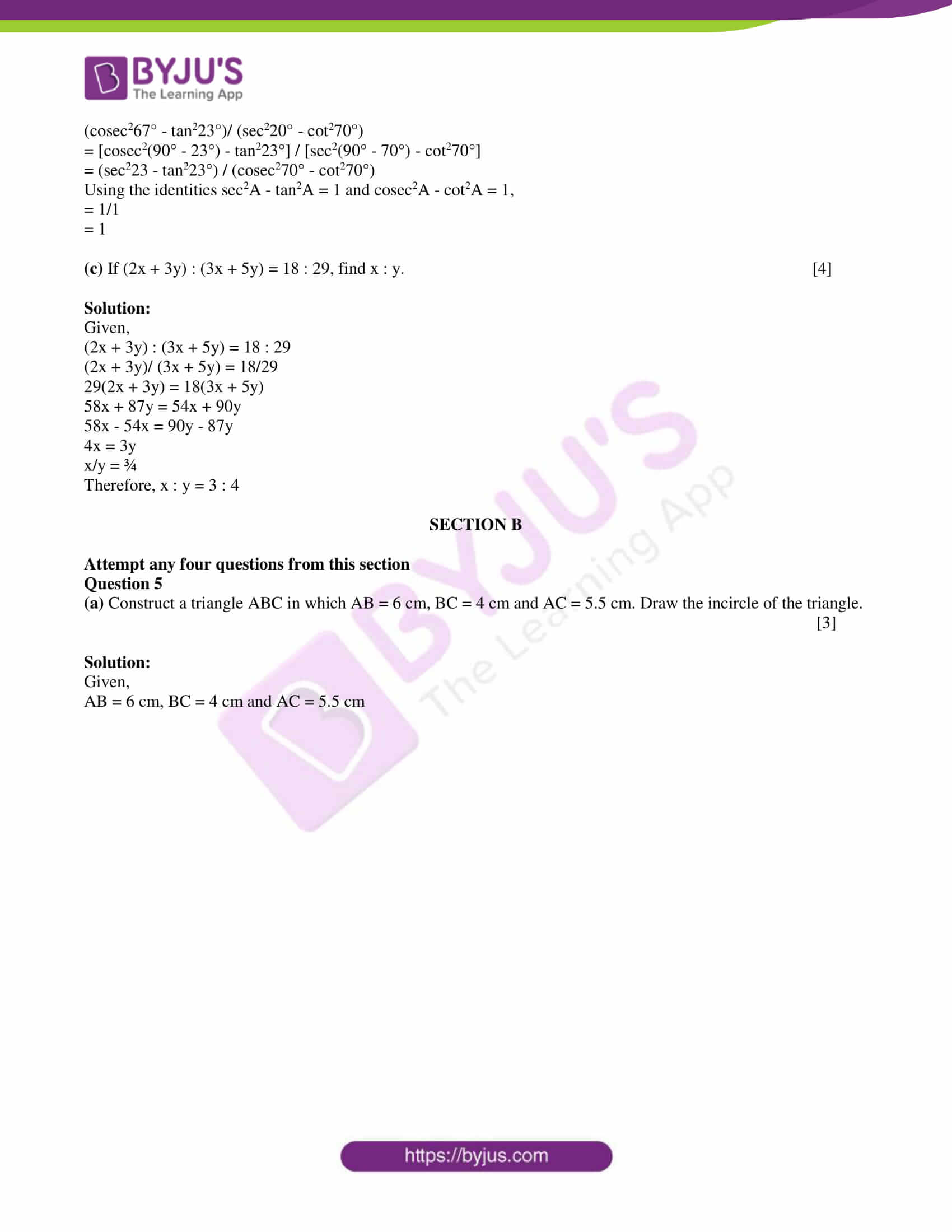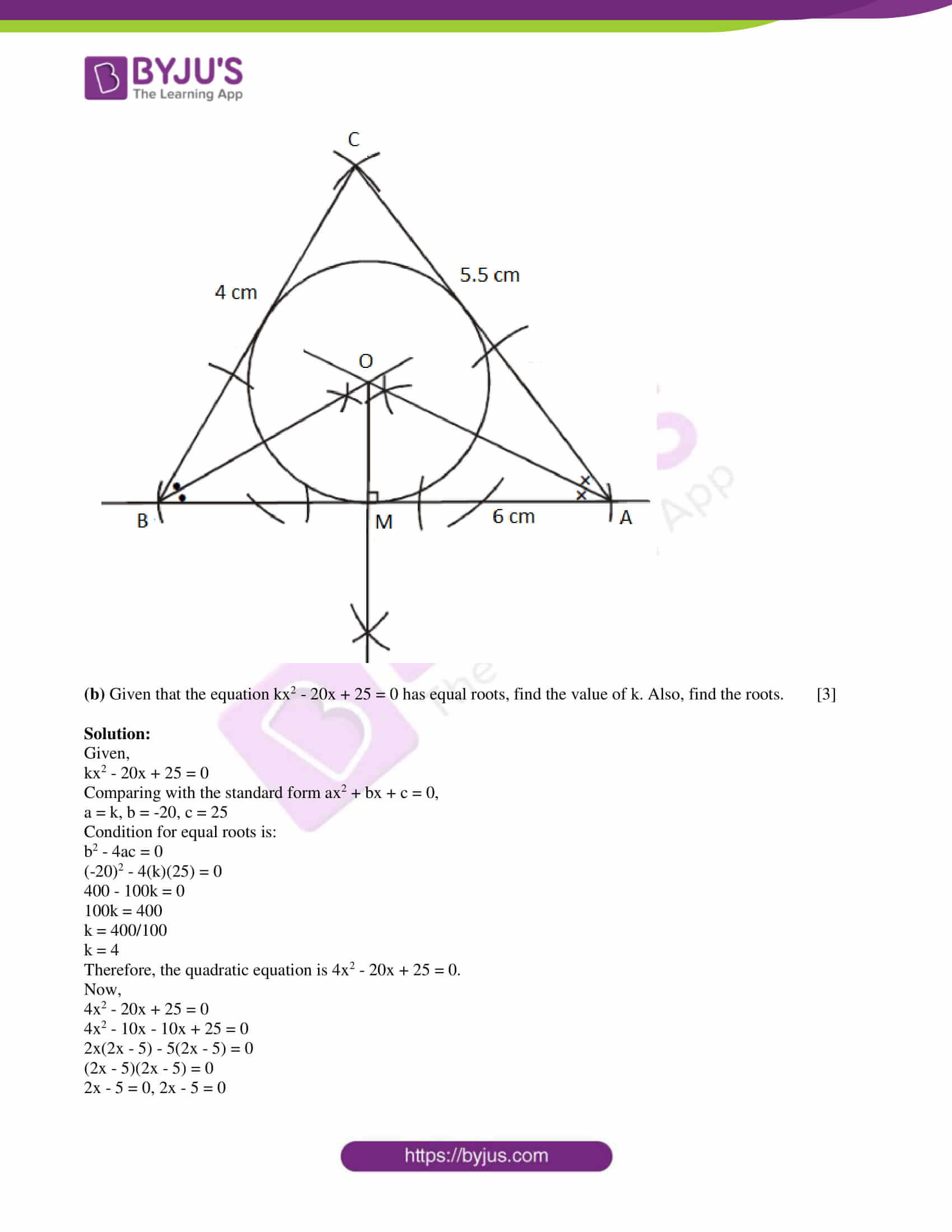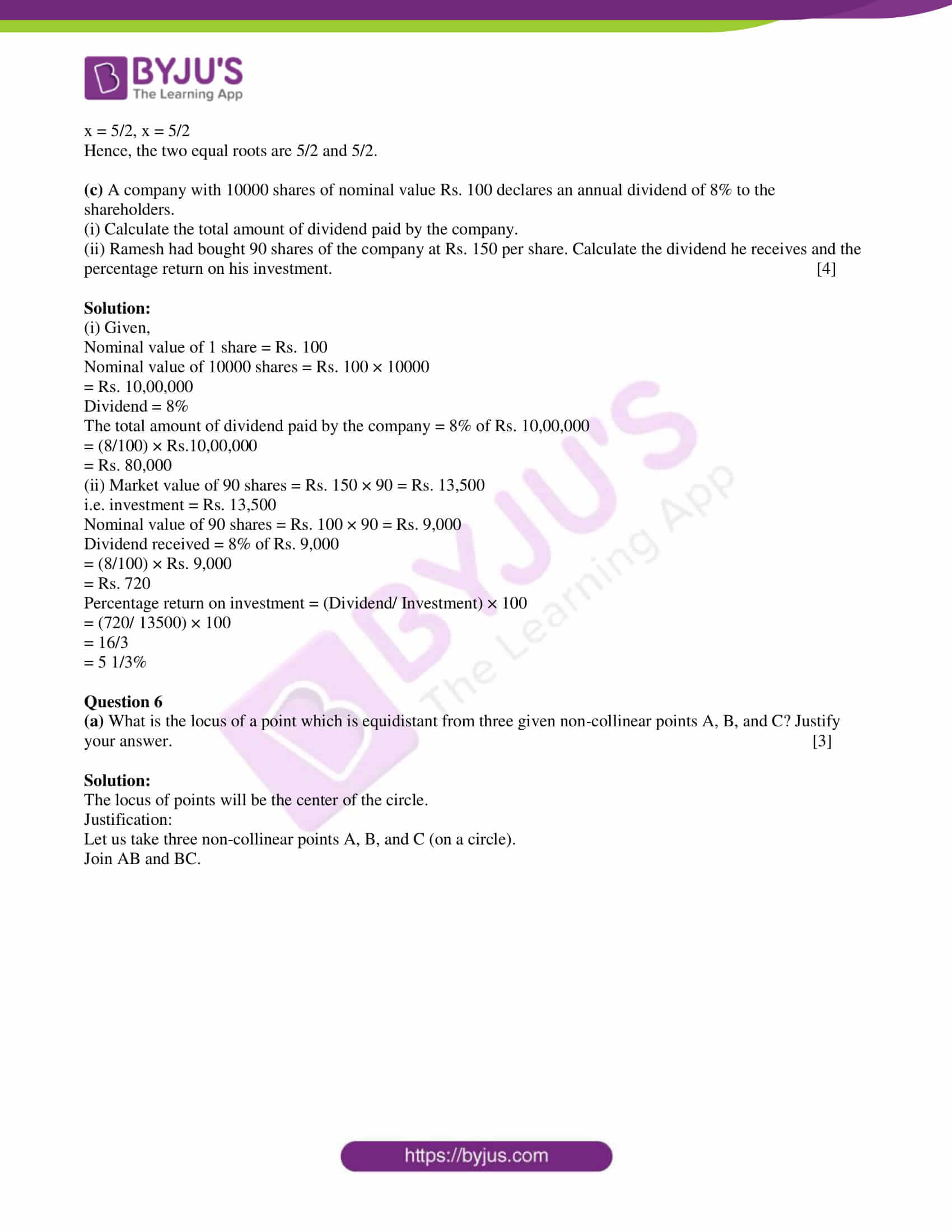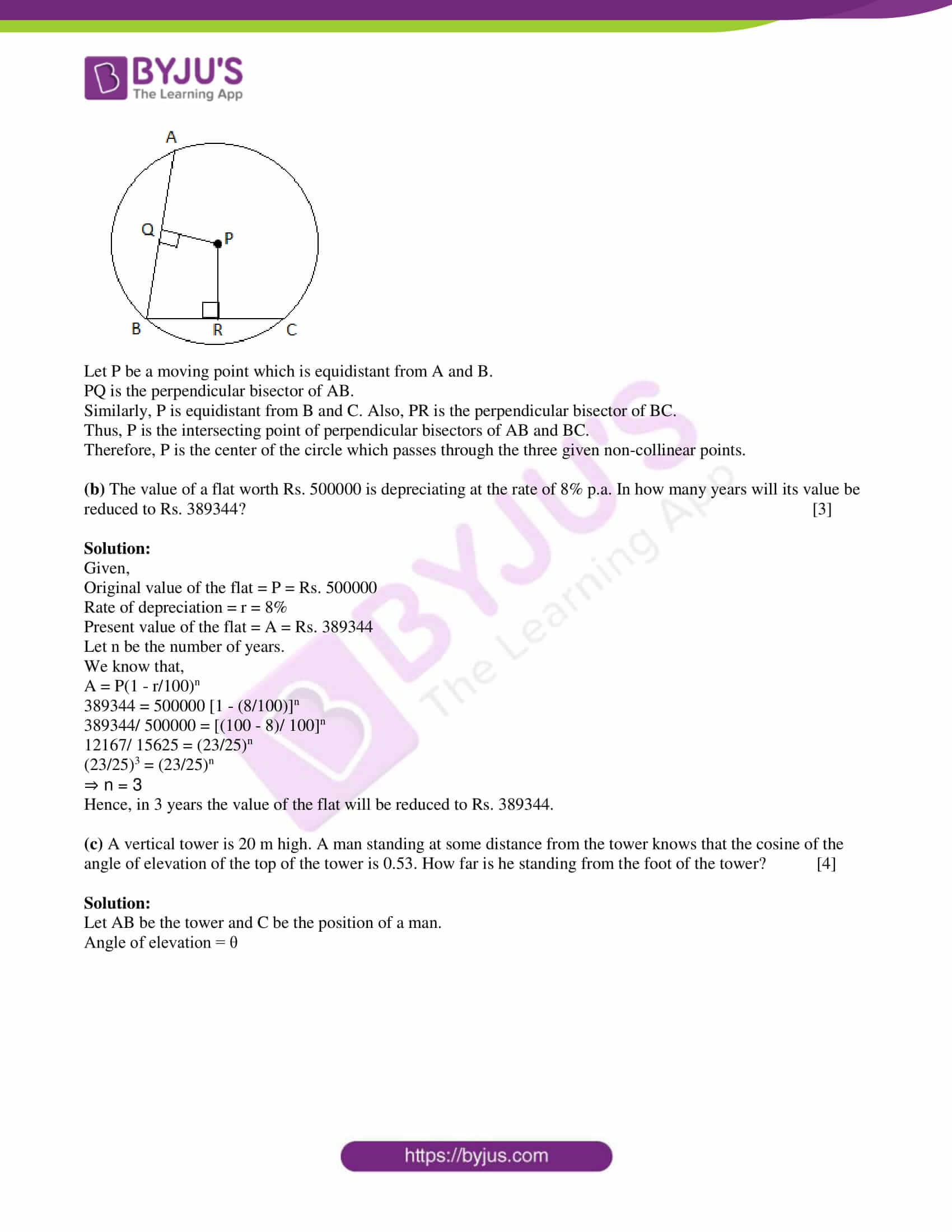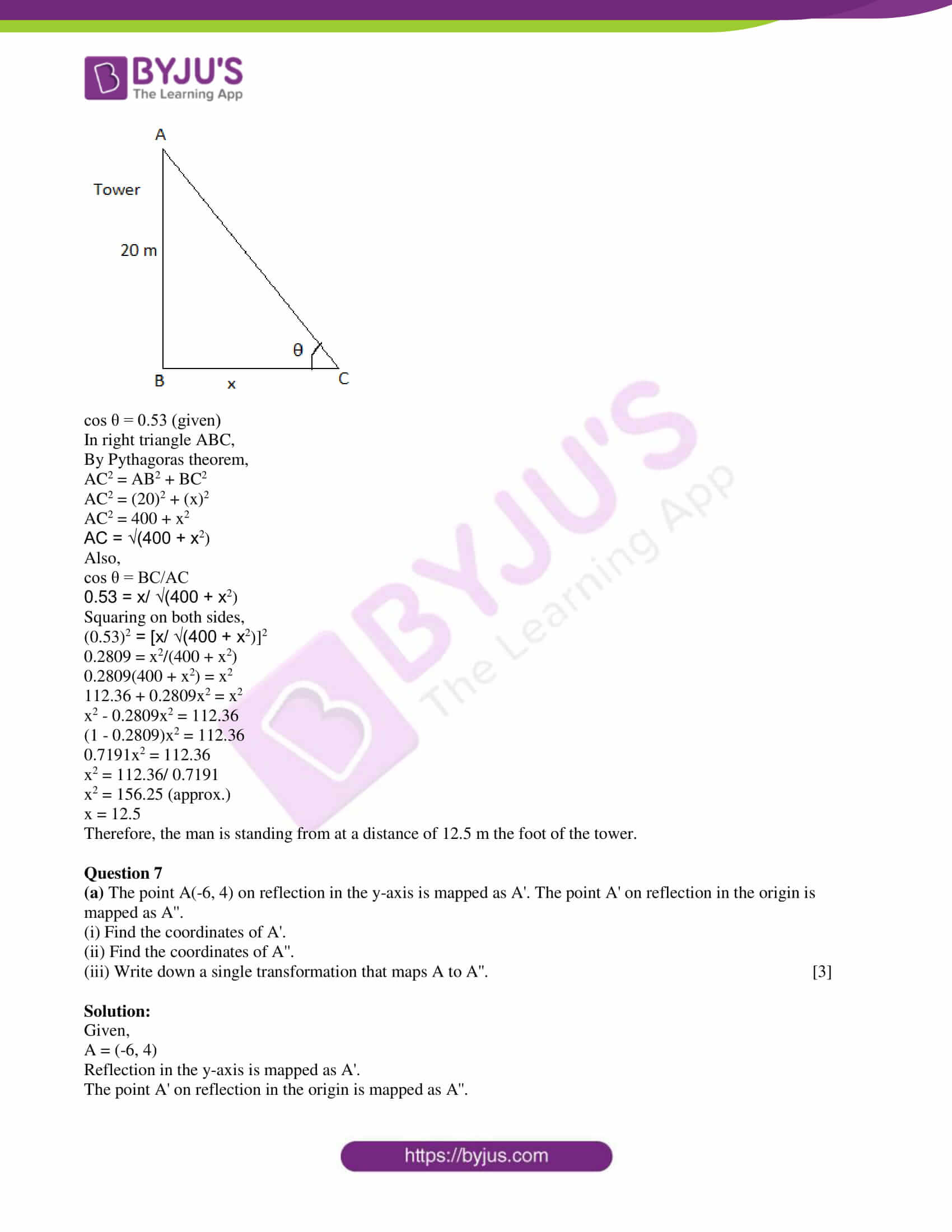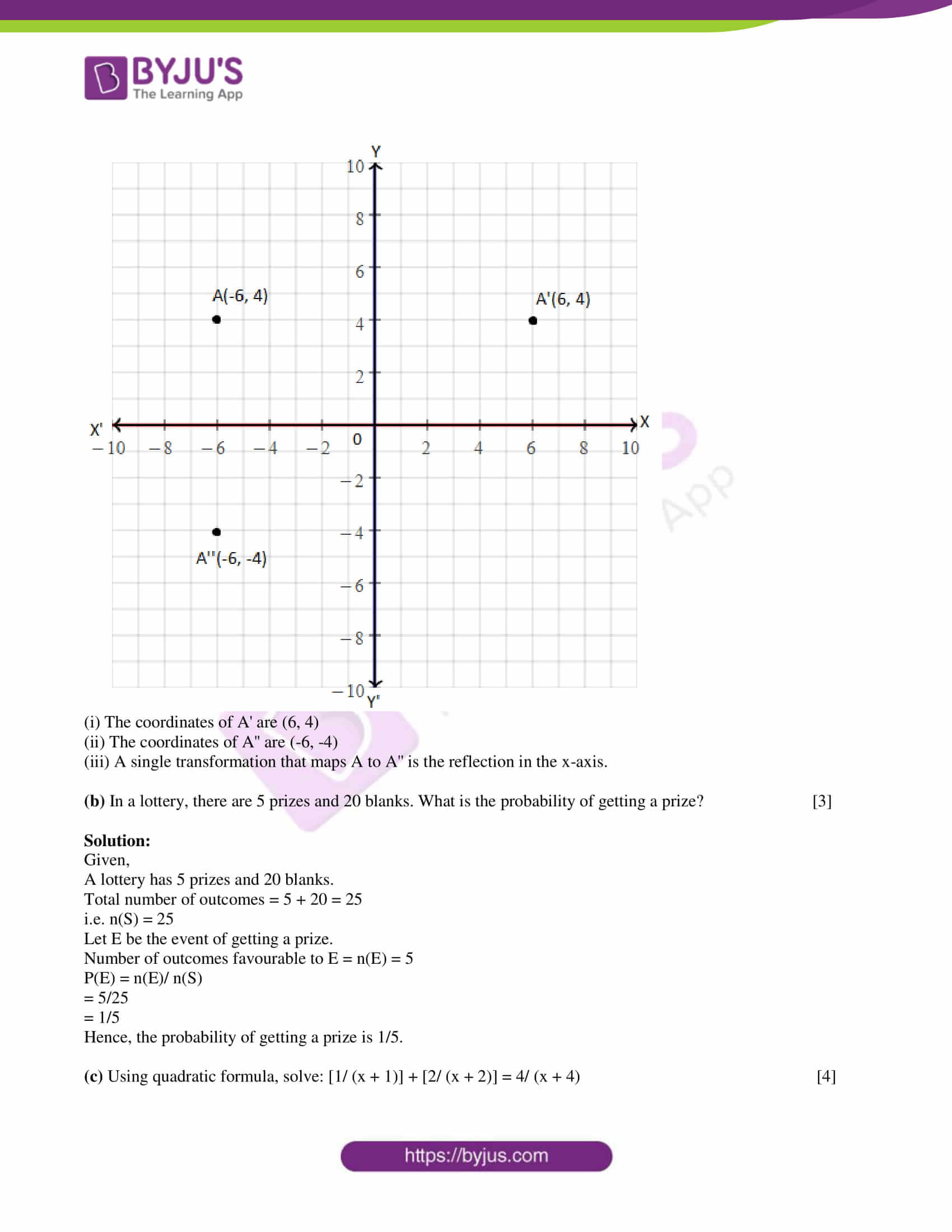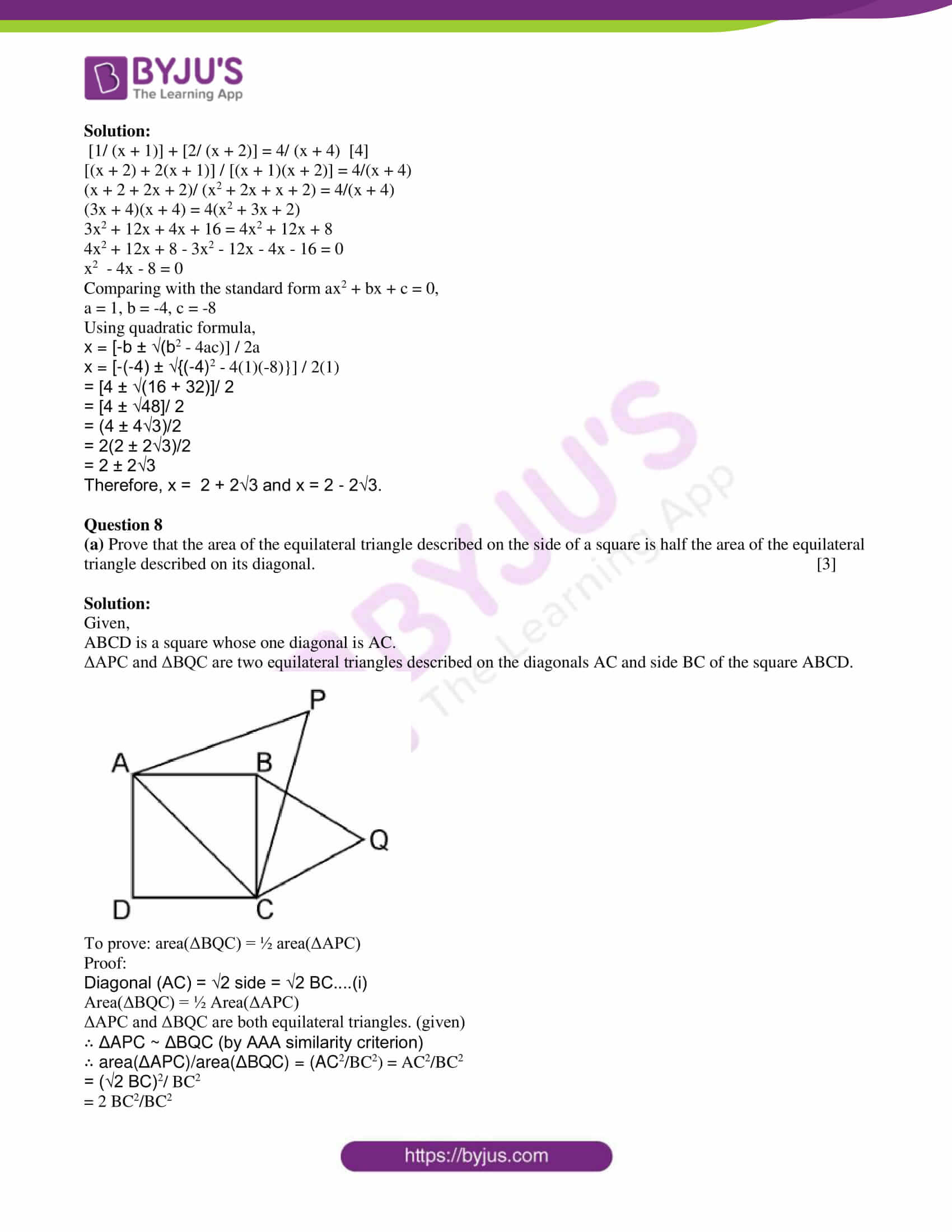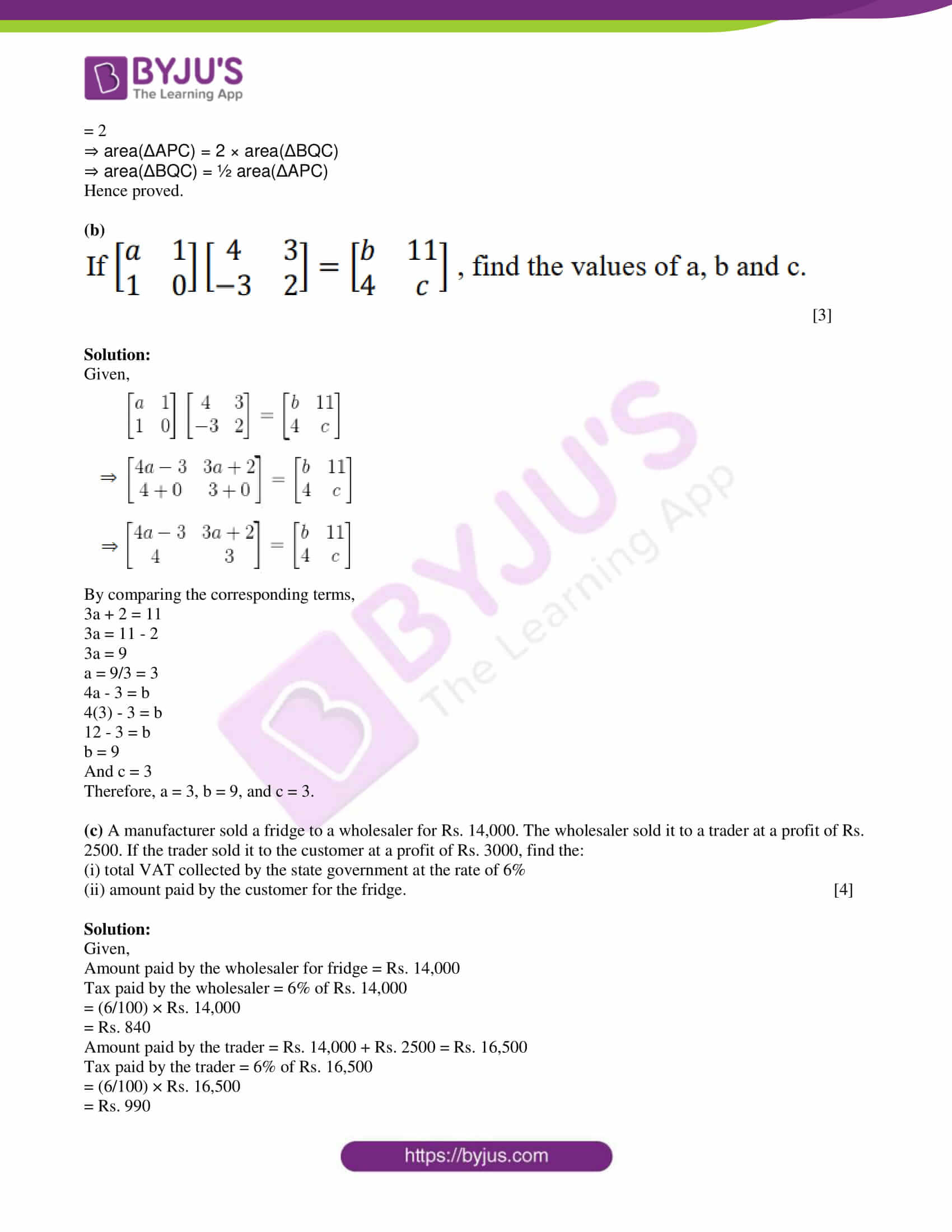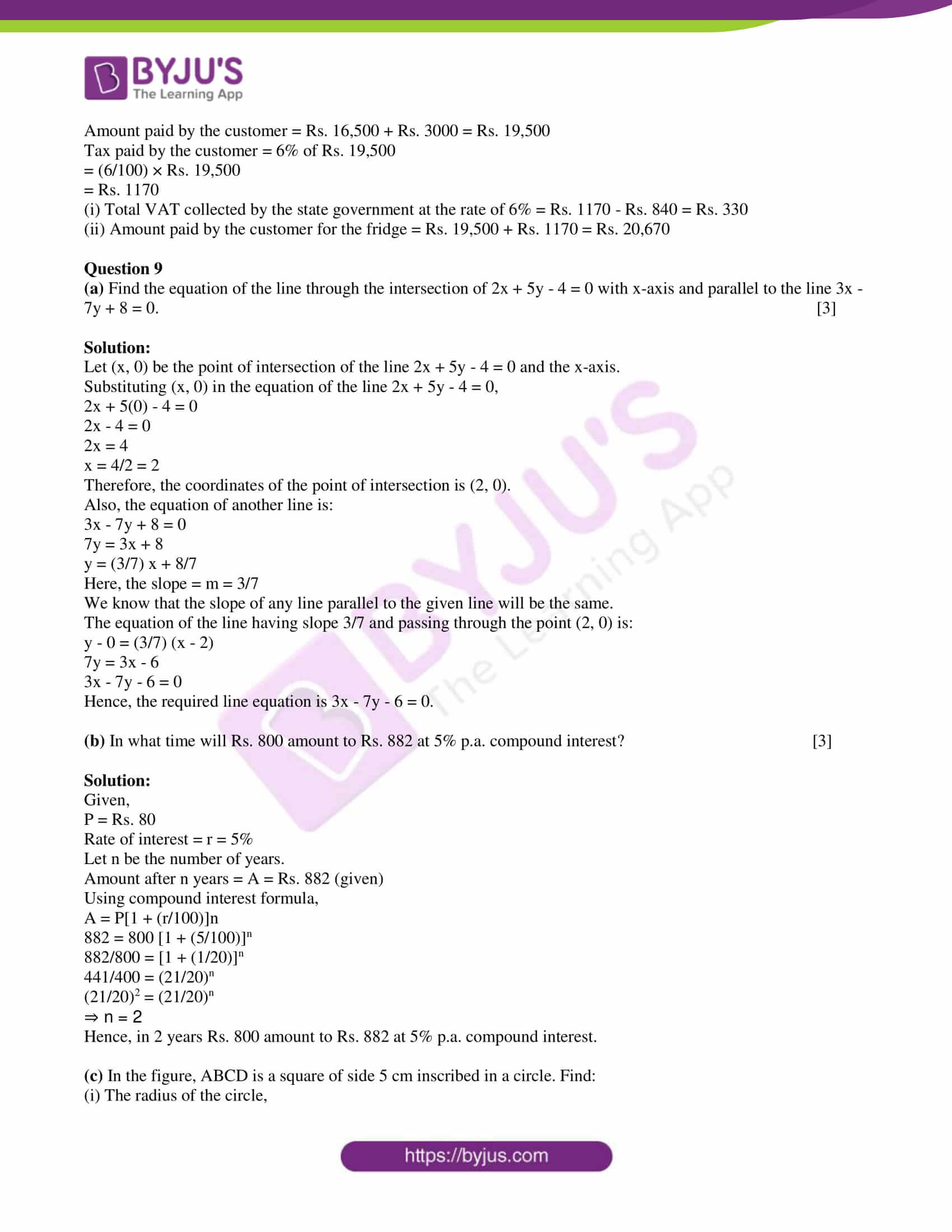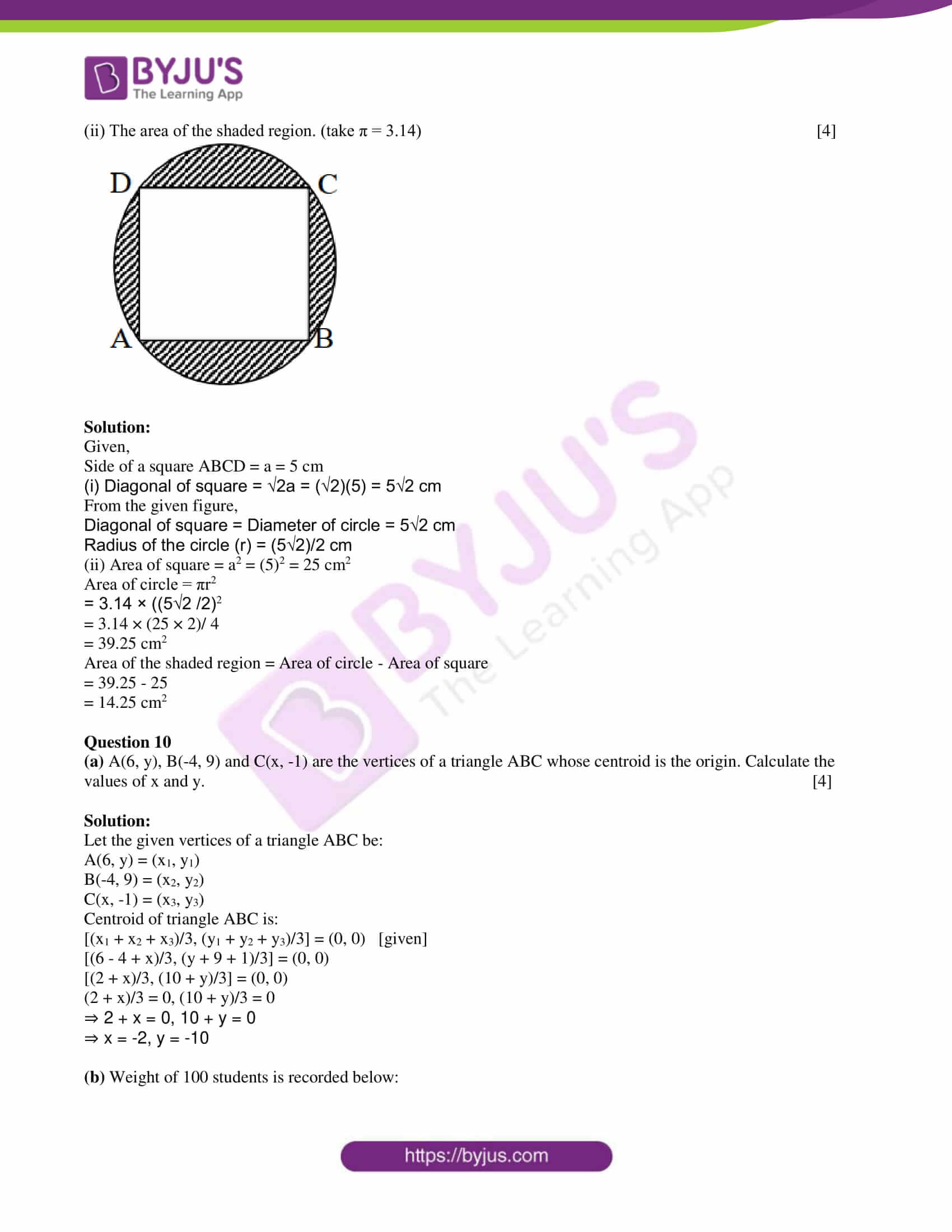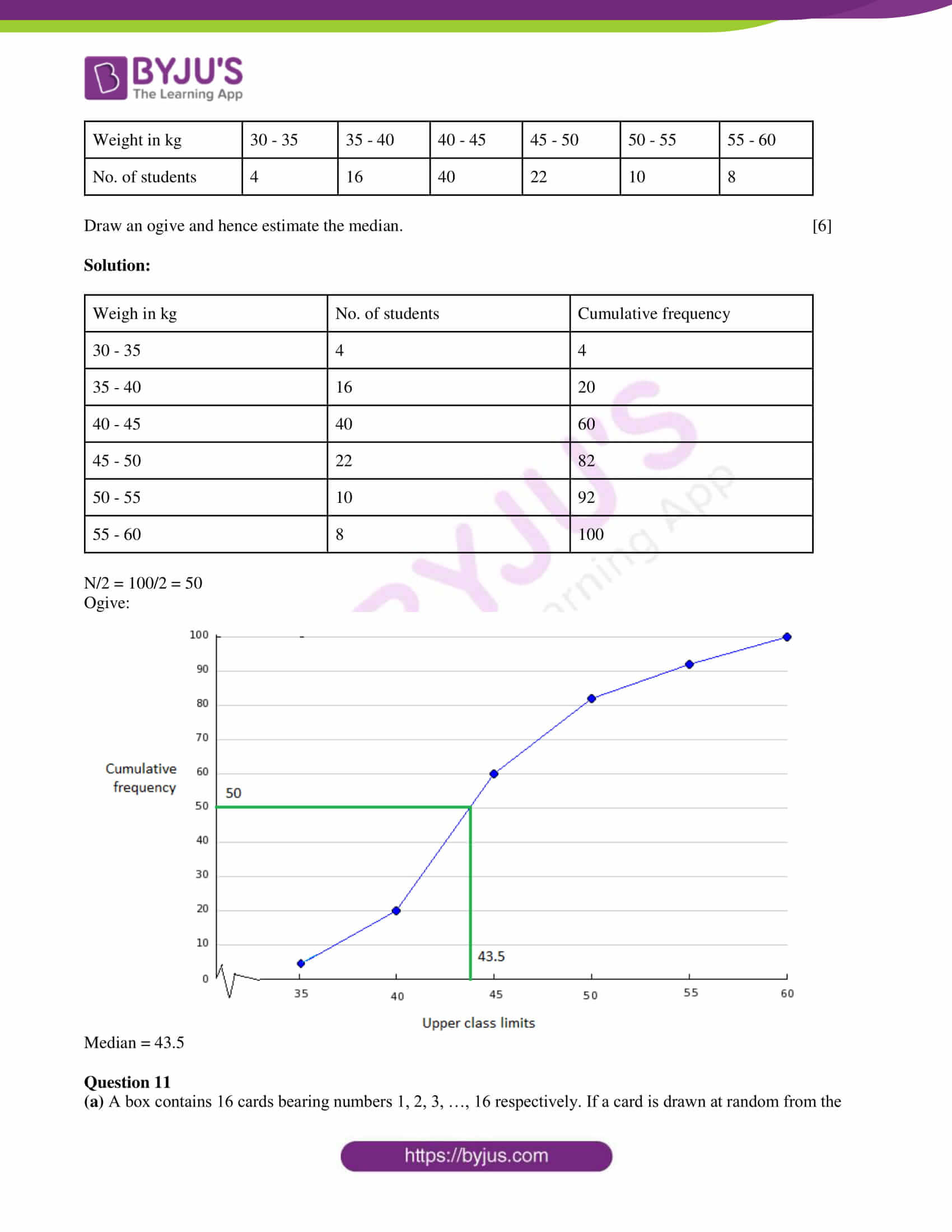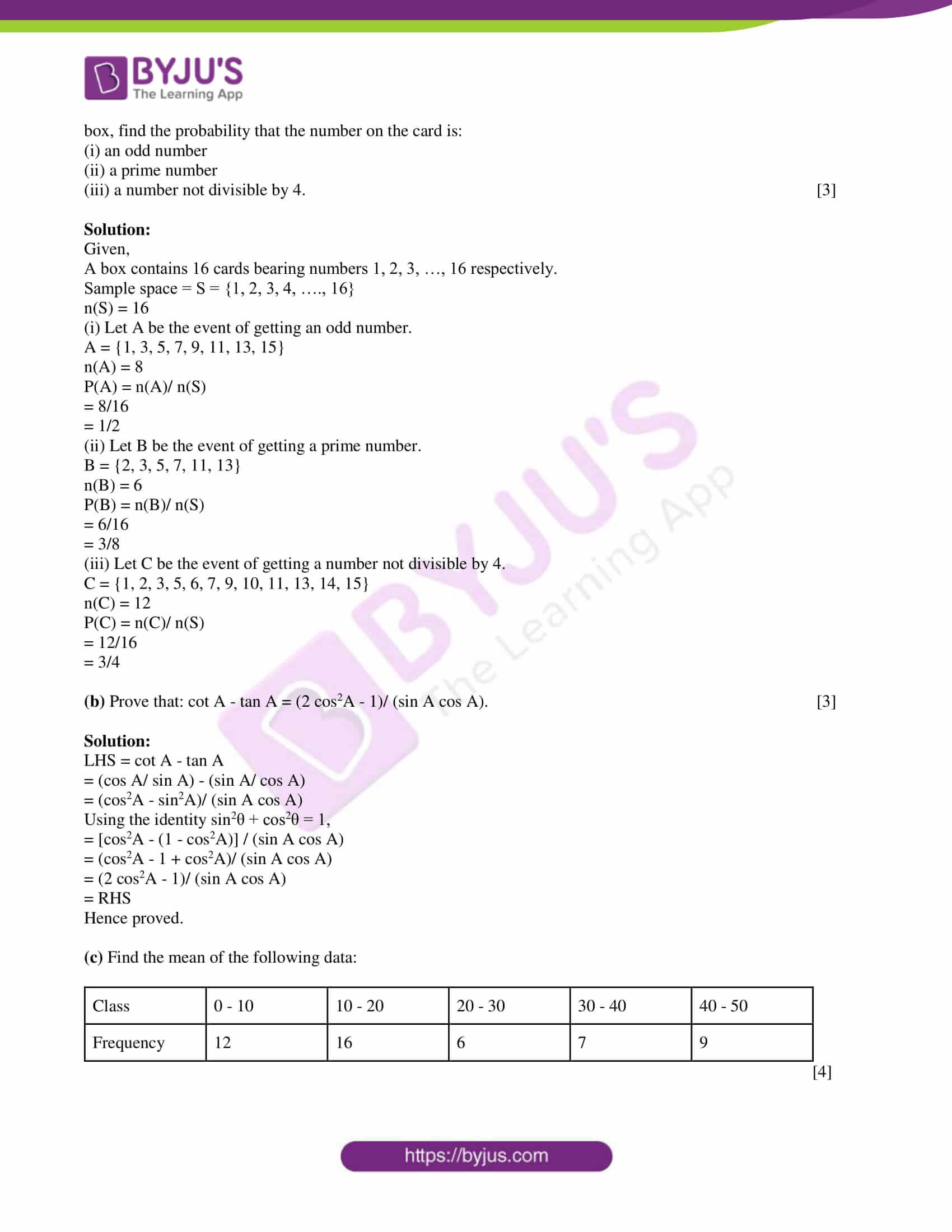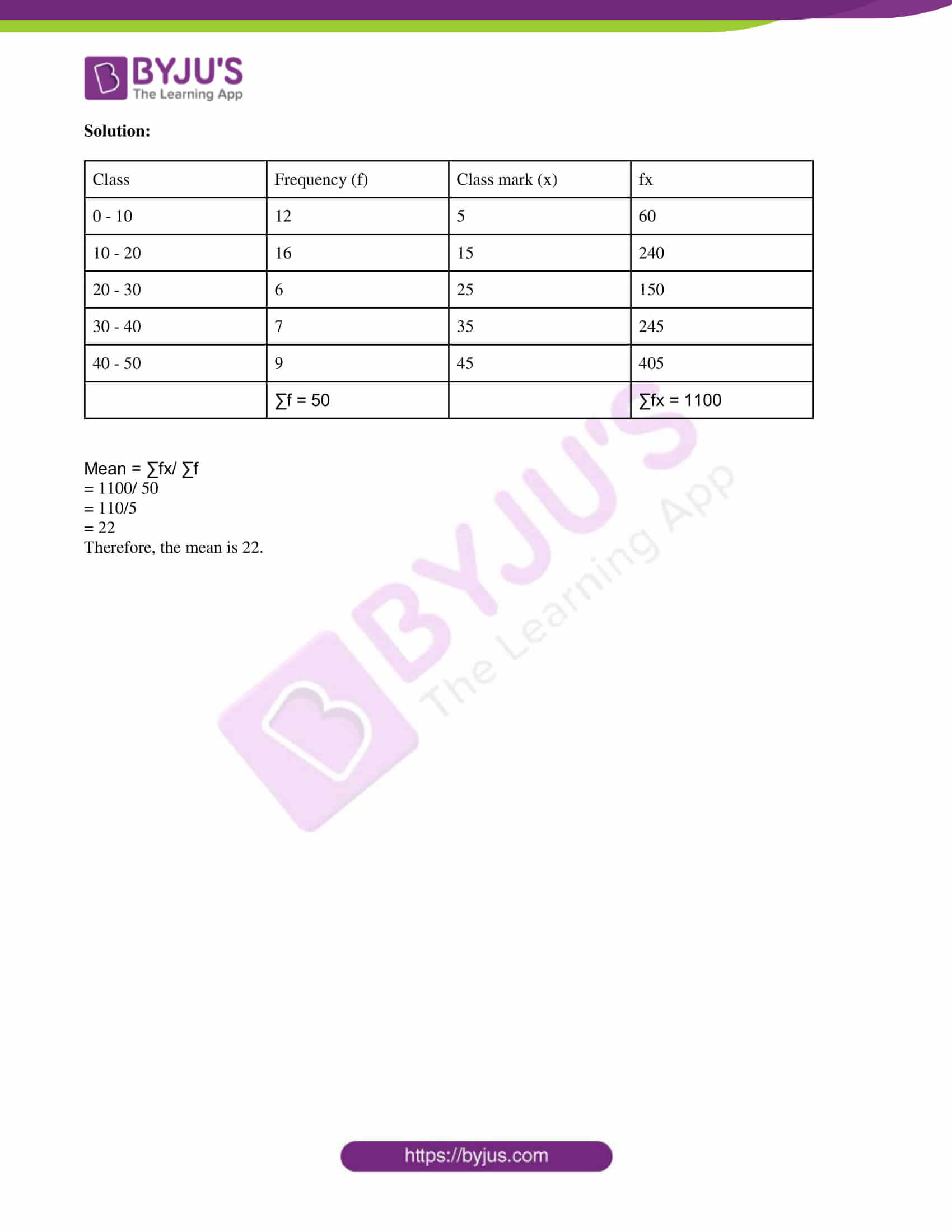SECTION A

Attempt all questions from this section.

Question 1

(a) Simplify: -10 ⅓ < (5y/3) + 3 < (y/2) + 5 ⅓, y ∈ R

Graph the solution set on the number line. 

Solution:

-10 ⅓ < (5y/3) + 3 < (y/2) + 5 ⅓

-31/3 < (5y/3) + 3 < (y/2) + 16/3

Now,

-31/3 < (5y/3) + 3

(-31/3) – 3 < 5y/3

(-40/3) < 5y/3

-40 < 5y

y > -40/5

y > -8

And

(5y/3) + 3 < (y/2) + 16/3

(5y/3) – (y/2) < (16/3) – 3

(10y – 3y)/ 6 < (16 – 9)/3

7y/6 < 7/3

y < 6/3

y < 2

Therefore, -8 < y < 2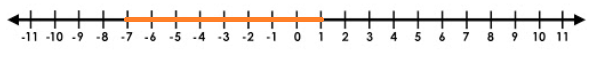(b) The polynomials (px3 + 3x2 – 3) and (2x3 – 5x + p) when divided by (x – 4) leave the same remainder. Find the value of p. 

Solution:

Let f(x) = px3 + 3x2 – 3

p(x) = 2x3 – 5x + p

Given that the polynomials f(x) and g(x) when divided by (x – 4) leave the same remainder.

Substituting x = 4 in f(x) and p(x),

f(4) = p(4)3 + 3(4)2 – 3

= 64p + 3(16) – 3

= 64p + 48 – 3

= 64p + 45

p(4) = 2(4)3 – 5(4) + p

= 2(64) – 20 + p

= 128 – 20 + p

= 108 + p

According to the given,

64p + 45 = 108 + p

64p – p = 108 – 45

63p = 63

p = 63/63

p = 1

(c)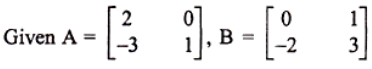Calculate: (i) 3A – 2B (ii) A2 – BA. 

Solution:

Given,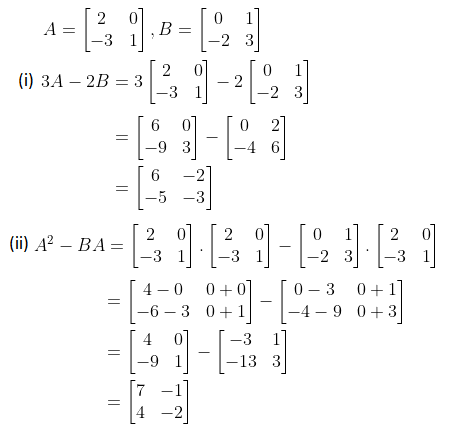Question 2

(a) The height of a cone is 5 cm. Find the height of another cone whose volume is sixteen times its volume and radius equal to its diameter. 

Solution:

Given,

Height of the first cone = h = 5 cm

Let r be the radius of the first cone and H be the height of the second cone.

The radius of the second cone = Diameter of the first cone

R = 2r

Given that the volume of the second cone is equal to the sixteen times the volume of the first cone.

(⅓)πR2H = 16 × (⅓)πr2h

(2r)2 × H = 16 × r2 × 5

4r2 × H = 80 × r2

H = 80/4

H = 20 cm

Hence, the height of the required cone is 20 cm.

(b) If a pair of opposite sides of a cyclic quadrilateral are equal, prove that its diagonals are equal. 

Solution:

Join AC and BD.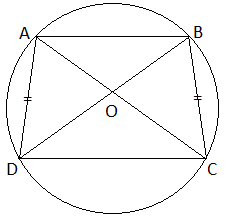To prove: AC = BD

In ΔAOD and ΔBOC,

We know that the same segment subtends an equal angle to the circle.

∠ODA = ∠OCB

AB = BC (from the given)

By ASA congruence criterion,

ΔAOD ≅ ΔBOC

ΔAOD+ ΔDOC ≅ ΔBOC + ΔDOC

AC = BD (by CPCT)

Hence probed.

(c) Rupa’s passbook has the following entries:

 Date 2009 Particulars Amount withdrawn (Rs) Amount deposited (Rs) Balance (Rs) Feb. 19 By cash _ 1000 1000 Feb. 25 By cash _ 2000 3000 March 1 By salary _ 5000 8000 March 10 To cheque 2000 _ 6000 March 27 To cheque 500 _ 5500 April 1 By salary _ 5000 10500

Find the amount received by him, if she closes her account on 11th April, when interest is 5% p.a. 

Solution:

From the given,

It is clear that Rupa will not receive interest for Feb and April.

Eligible amount for interest (i.e. March) = Rs. 5500

Rate of interest = R = 5%

SI = PTR/100

= (5500 × 1 × 5)/ (12 × 100)

= Rs. 22.917

She closed the account on 11th April.

The amount available by the time of closing her account = Rs. 10500

Amount received by Rupa = Rs. 10500 + Rs. 22.917

= Rs. 10522.917

Question 3

(a) In the given figure, AB is a diameter of the circle with centre O and ∠OAT = 90° and C is a point on the circle. Calculate the numerical value of x.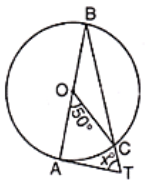Solution:

Given,

AB is a diameter of the circle with a centre O and ∠OAT = 90°.

C is a point on the circle.

∠AOC = 50°

We know that the angle subtended by an arc of the circle at the centre is twice the angle subtended by it at any point in the remaining part of the circle.

∠AOC = 2∠ABC

∠ABC = (½) ∠AOC

= (½) × 50°

= 25°

Thus, ∠ABC = ∠ABT = 25°

And ∠OAT = ∠BAT = 90°

In triangle BAT,

∠BAT + ∠ABT + ∠ATB = 180° (by the angle sum property)

90° + 25° + x = 180°

x = 180° – 90° – 25°

x = 65°

(b) If the mean of 10 observations is 20 and that of another 15 observations is 16, find the mean of all 25 observations. 

Solution:

Given,

Mean of 10 observations = 20

Sum of 10 observations = 20 × 10 = 200

And

The mean of 15 observations = 16

Sum of 15 observations = 16 × 15 = 240

Sum of all the 25 observations = 200 + 240 = 440

Mean of 25 observations = 440/ 25

= 17.6

(c) On a graph paper plot the points A(-2, 0), B(4, 0), C(1, 4), D(-2, 4) and E(4, 4). Give the specific name to quadrilateral ABED.

Name the common line of symmetry of ΔABC and quadrilateral ABED. 

Solution:

Given,

A(-2, 0), B(4, 0), C(1, 4), D(-2, 4) and E(4, 4).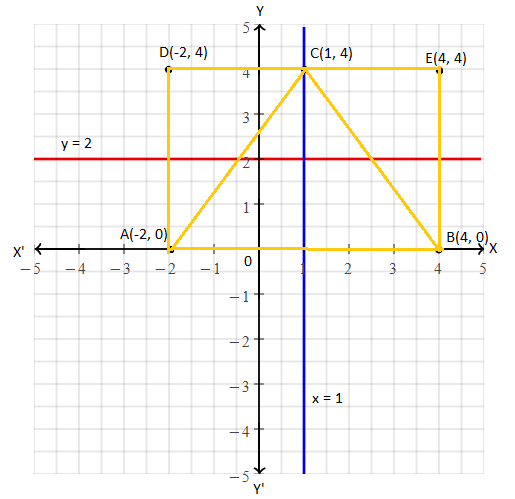The specific name of a quadrilateral ABED is a rectangle.

The common line of symmetry of ΔABC and quadrilateral ABED is x = 1 and y = 2.

Question 4

(a) Show that (3x – 1) is a factor of 6x2 + 7x – 3. 

Solution:

Let p(x) = 6x2 + 7x – 3

For checking (3x – 1) is a factor of p(x) or not, substitute x = 1/3 in p(x),

p(1/3) = 6(⅓)2 + 7(⅓) – 3

= 6(1/9) + (7/3) – 3

= (⅔) + (7/3) – 3

= (2 + 7)/3 – 3

= (9/3) – 3

= 3 – 3

= 0

Therefore, (3x – 1) is not a factor of the given polynomial 6x2 + 7x – 3.

(b) Without using tables, find the value of (cosec267° – tan223°)/ (sec220° – cot270°). 

Solution:

(cosec267° – tan223°)/ (sec220° – cot270°)

= [cosec2(90° – 23°) – tan223°] / [sec2(90° – 70°) – cot270°]

= (sec223 – tan223°) / (cosec270° – cot270°)

Using the identities sec2A – tan2A = 1 and cosec2A – cot2A = 1,

= 1/1

= 1

(c) If (2x + 3y) : (3x + 5y) = 18 : 29, find x : y. 

Solution:

Given,

(2x + 3y) : (3x + 5y) = 18 : 29

(2x + 3y)/ (3x + 5y) = 18/29

29(2x + 3y) = 18(3x + 5y)

58x + 87y = 54x + 90y

58x – 54x = 90y – 87y

4x = 3y

x/y = ¾

Therefore, x : y = 3 : 4

SECTION B

Attempt any four questions from this section

Question 5

(a) Construct a triangle ABC in which AB = 6 cm, BC = 4 cm and AC = 5.5 cm. Draw the incircle of the triangle. 

Solution:

Given,

AB = 6 cm, BC = 4 cm and AC = 5.5 cm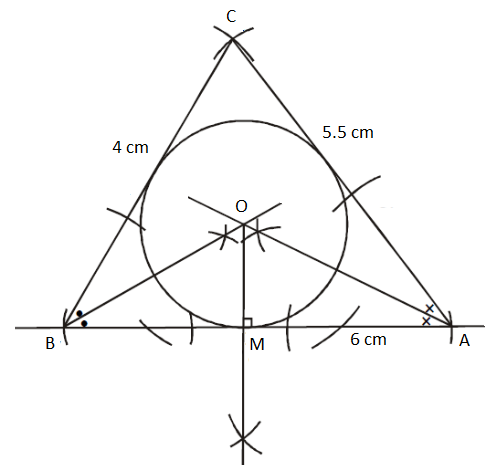(b) Given that the equation kx2 – 20x + 25 = 0 has equal roots, find the value of k. Also, find the roots. 

Solution:

Given,

kx2 – 20x + 25 = 0

Comparing with the standard form ax2 + bx + c = 0,

a = k, b = -20, c = 25

Condition for equal roots is:

b2 – 4ac = 0

(-20)2 – 4(k)(25) = 0

400 – 100k = 0

100k = 400

k = 400/100

k = 4

Therefore, the quadratic equation is 4x2 – 20x + 25 = 0.

Now,

4x2 – 20x + 25 = 0

4x2 – 10x – 10x + 25 = 0

2x(2x – 5) – 5(2x – 5) = 0

(2x – 5)(2x – 5) = 0

2x – 5 = 0, 2x – 5 = 0

x = 5/2, x = 5/2

Hence, the two equal roots are 5/2 and 5/2.

(c) A company with 10000 shares of nominal value Rs. 100 declares an annual dividend of 8% to the shareholders.

(i) Calculate the total amount of dividend paid by the company.

(ii) Ramesh had bought 90 shares of the company at Rs. 150 per share. Calculate the dividend he receives and the percentage return on his investment. 

Solution:

(i) Given,

Nominal value of 1 share = Rs. 100

Nominal value of 10000 shares = Rs. 100 × 10000

= Rs. 10,00,000

Dividend = 8%

The total amount of dividend paid by the company = 8% of Rs. 10,00,000

= (8/100) × Rs.10,00,000

= Rs. 80,000

(ii) Market value of 90 shares = Rs. 150 × 90 = Rs. 13,500

i.e. investment = Rs. 13,500

Nominal value of 90 shares = Rs. 100 × 90 = Rs. 9,000

Dividend received = 8% of Rs. 9,000

= (8/100) × Rs. 9,000

= Rs. 720

Percentage return on investment = (Dividend/ Investment) × 100

= (720/ 13500) × 100

= 16/3

= 5 1/3%

Question 6

(a) What is the locus of a point which is equidistant from three given non-collinear points A, B, and C? Justify your answer. 

Solution:

The locus of points will be the center of the circle.

Justification:

Let us take three non-collinear points A, B, and C (on a circle).

Join AB and BC.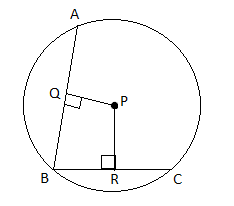Let P be a moving point which is equidistant from A and B.

PQ is the perpendicular bisector of AB.

Similarly, P is equidistant from B and C. Also, PR is the perpendicular bisector of BC.

Thus, P is the intersecting point of perpendicular bisectors of AB and BC.

Therefore, P is the center of the circle which passes through the three given non-collinear points.

(b) The value of a flat worth Rs. 500000 is depreciating at the rate of 8% p.a. In how many years will its value be reduced to Rs. 389344? 

Solution:

Given,

Original value of the flat = P = Rs. 500000

Rate of depreciation = r = 8%

Present value of the flat = A = Rs. 389344

Let n be the number of years.

We know that,

A = P(1 – r/100)n

389344 = 500000 [1 – (8/100)]n

389344/ 500000 = [(100 – 8)/ 100]n

12167/ 15625 = (23/25)n

(23/25)3 = (23/25)n

⇒ n = 3

Hence, in 3 years the value of the flat will be reduced to Rs. 389344.

(c) A vertical tower is 20 m high. A man standing at some distance from the tower knows that the cosine of the angle of elevation of the top of the tower is 0.53. How far is he standing from the foot of the tower? 

Solution:

Let AB be the tower and C be the position of a man.

Angle of elevation = θ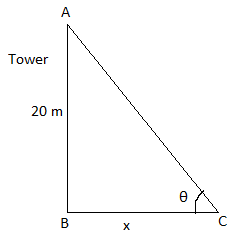cos θ = 0.53 (given)

In right triangle ABC,

By Pythagoras theorem,

AC2 = AB2 + BC2

AC2 = (20)2 + (x)2

AC2 = 400 + x2

AC = √(400 + x2)

Also,

cos θ = BC/AC

0.53 = x/ √(400 + x2)

Squaring on both sides,

(0.53)2 = [x/ √(400 + x2)]2

0.2809 = x2/(400 + x2)

0.2809(400 + x2) = x2

112.36 + 0.2809x2 = x2

x2 – 0.2809x2 = 112.36

(1 – 0.2809)x2 = 112.36

0.7191x2 = 112.36

x2 = 112.36/ 0.7191

x2 = 156.25 (approx)

x = 12.5

Therefore, the man is standing from at a distance of 12.5 m the foot of the tower.

Question 7

(a) The point A(-6, 4) on reflection in the y-axis is mapped as A’. The point A’ on reflection in the origin is mapped as A”.

(i) Find the coordinates of A’.

(ii) Find the coordinates of A”.

(iii) Write down a single transformation that maps A to A”. 

Solution:

Given,

A = (-6, 4)

Reflection in the y-axis is mapped as A’.

The point A’ on reflection in the origin is mapped as A”.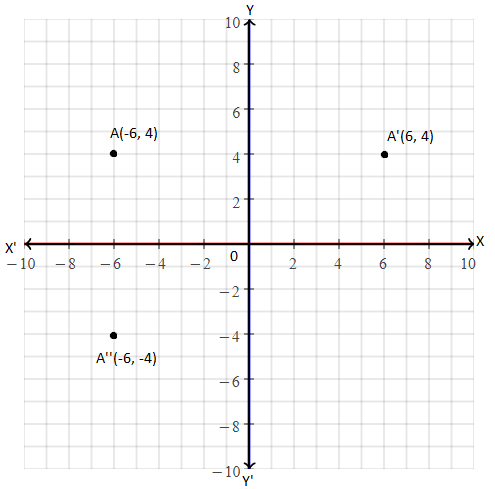(i) The coordinates of A’ are (6, 4)

(ii) The coordinates of A” are (-6, -4)

(iii) A single transformation that maps A to A” is the reflection in the x-axis.

(b) In a lottery, there are 5 prizes and 20 blanks. What is the probability of getting a prize? 

Solution:

Given,

A lottery has 5 prizes and 20 blanks.

Total number of outcomes = 5 + 20 = 25

i.e. n(S) = 25

Let E be the event of getting a prize.

Number of outcomes favourable to E = n(E) = 5

P(E) = n(E)/ n(S)

= 5/25

= 1/5

Hence, the probability of getting a prize is 1/5.

(c) Using quadratic formula, solve: [1/ (x + 1)] + [2/ (x + 2)] = 4/ (x + 4) 

Solution:

[1/ (x + 1)] + [2/ (x + 2)] = 4/ (x + 4)  [(x + 2) + 2(x + 1)] / [(x + 1)(x + 2)] = 4/(x + 4)

(x + 2 + 2x + 2)/ (x2 + 2x + x + 2) = 4/(x + 4)

(3x + 4)(x + 4) = 4(x2 + 3x + 2)

3x2 + 12x + 4x + 16 = 4x2 + 12x + 8

4x2 + 12x + 8 – 3x2 – 12x – 4x – 16 = 0

x2 – 4x – 8 = 0

Comparing with the standard form ax2 + bx + c = 0,

a = 1, b = -4, c = -8

x = [-b ± √(b2 – 4ac)] / 2a

x = [-(-4) ± √{(-4)2 – 4(1)(-8)}] / 2(1)

= [4 ± √(16 + 32)]/ 2

= [4 ± √48]/ 2

= (4 ± 4√3)/2

= 2(2 ± 2√3)/2

= 2 ± 2√3

Therefore, x = 2 + 2√3 and x = 2 – 2√3.

Question 8

(a) Prove that the area of the equilateral triangle described on the side of a square is half the area of the equilateral triangle described on its diagonal. 

Solution:

Given,

ABCD is a square whose one diagonal is AC.

ΔAPC and ΔBQC are two equilateral triangles described on the diagonals AC and side BC of the square ABCD.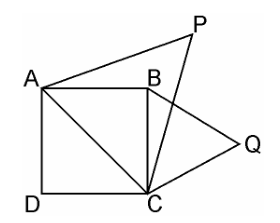To prove: area(ΔBQC) = ½ area(ΔAPC)

Proof:

Diagonal (AC) = √2 side = √2 BC….(i)

Area(ΔBQC) = ½ Area(ΔAPC)

ΔAPC and ΔBQC are both equilateral triangles. (given)

∴ ΔAPC ~ ΔBQC (by AAA similarity criterion)

∴ area(ΔAPC)/area(ΔBQC) = (AC2/BC2) = AC2/BC2

= (√2 BC)2/ BC2

= 2 BC2/BC2

= 2

⇒ area(ΔAPC) = 2 × area(ΔBQC)

⇒ area(ΔBQC) = ½ area(ΔAPC)

Hence proved.

(b)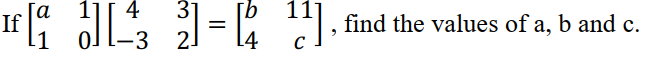Solution:

Given,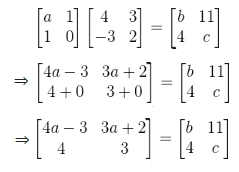By comparing the corresponding terms,

3a + 2 = 11

3a = 11 – 2

3a = 9

a = 9/3 = 3

4a – 3 = b

4(3) – 3 = b

12 – 3 = b

b = 9

And c = 3

Therefore, a = 3, b = 9, and c = 3.

(c) A manufacturer sold a fridge to a wholesaler for Rs. 14,000. The wholesaler sold it to a trader at a profit of Rs. 2500. If the trader sold it to the customer at a profit of Rs. 3000, find the:

(i) total VAT collected by the state government at the rate of 6%

(ii) amount paid by the customer for the fridge. 

Solution:

Given,

Amount paid by the wholesaler for fridge = Rs. 14,000

Tax paid by the wholesaler = 6% of Rs. 14,000

= (6/100) × Rs. 14,000

= Rs. 840

Amount paid by the trader = Rs. 14,000 + Rs. 2500 = Rs. 16,500

Tax paid by the trader = 6% of Rs. 16,500

= (6/100) × Rs. 16,500

= Rs. 990

Amount paid by the customer = Rs. 16,500 + Rs. 3000 = Rs. 19,500

Tax paid by the customer = 6% of Rs. 19,500

= (6/100) × Rs. 19,500

= Rs. 1170

(i) Total VAT collected by the state government at the rate of 6% = Rs. 1170 – Rs. 840 = Rs. 330

(ii) Amount paid by the customer for the fridge = Rs. 19,500 + Rs. 1170 = Rs. 20,670

Question 9

(a) Find the equation of the line through the intersection of 2x + 5y – 4 = 0 with x-axis and parallel to the line 3x – 7y + 8 = 0. 

Solution:

Let (x, 0) be the point of intersection of the line 2x + 5y – 4 = 0 and the x-axis.

Substituting (x, 0) in the equation of the line 2x + 5y – 4 = 0,

2x + 5(0) – 4 = 0

2x – 4 = 0

2x = 4

x = 4/2 = 2

Therefore, the coordinates of the point of intersection is (2, 0).

Also, the equation of another line is:

3x – 7y + 8 = 0

7y = 3x + 8

y = (3/7) x + 8/7

Here, the slope = m = 3/7

We know that the slope of any line parallel to the given line will be the same.

The equation of the line having slope 3/7 and passing through the point (2, 0) is:

y – 0 = (3/7) (x – 2)

7y = 3x – 6

3x – 7y – 6 = 0

Hence, the required line equation is 3x – 7y – 6 = 0.

(b) In what time will Rs. 800 amount to Rs. 882 at 5% p.a. compound interest? 

Solution:

Given,

P = Rs. 80

Rate of interest = r = 5%

Let n be the number of years.

Amount after n years = A = Rs. 882 (given)

Using compound interest formula,

A = P[1 + (r/100)]n

882 = 800 [1 + (5/100)]n

882/800 = [1 + (1/20)]n

441/400 = (21/20)n

(21/20)2 = (21/20)n

⇒ n = 2

Hence, in 2 years Rs. 800 amount to Rs. 882 at 5% p.a. compound interest.

(c) In the figure, ABCD is a square of side 5 cm inscribed in a circle. Find:

(i) The radius of the circle,

(ii) The area of the shaded region. (take π = 3.14)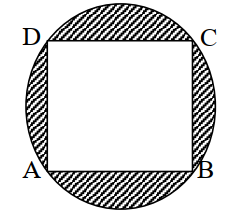Solution:

Given,

Side of a square ABCD = a = 5 cm

(i) Diagonal of square = √2a = (√2)(5) = 5√2 cm

From the given figure,

Diagonal of square = Diameter of circle = 5√2 cm

Radius of the circle (r) = (5√2)/2 cm

(ii) Area of square = a2 = (5)2 = 25 cm2

Area of circle = πr2

= 3.14 × ((5√2 /2)2

= 3.14 × (25 × 2)/ 4

= 39.25 cm2

Area of the shaded region = Area of circle – Area of square

= 39.25 – 25

= 14.25 cm2

Question 10

(a) A(6, y), B(-4, 9) and C(x, -1) are the vertices of a triangle ABC whose centroid is the origin. Calculate the values of x and y. 

Solution:

Let the given vertices of a triangle ABC be:

A(6, y) = (x1, y1)

B(-4, 9) = (x2, y2)

C(x, -1) = (x3, y3)

Centroid of triangle ABC is:

[(x1 + x2 + x3)/3, (y1 + y2 + y3)/3] = (0, 0) [given] [(6 – 4 + x)/3, (y + 9 + 1)/3] = (0, 0)

[(2 + x)/3, (10 + y)/3] = (0, 0)

(2 + x)/3 = 0, (10 + y)/3 = 0

⇒ 2 + x = 0, 10 + y = 0

⇒ x = -2, y = -10

(b) Weight of 100 students is recorded below:

 Weight in kg 30 – 35 35 – 40 40 – 45 45 – 50 50 – 55 55 – 60 No. of students 4 16 40 22 10 8

Draw an ogive and hence estimate the median. 

Solution:

 Weight in kg No. of students Cumulative frequency 30 – 35 4 4 35 – 40 16 20 40 – 45 40 60 45 – 50 22 82 50 – 55 10 92 55 – 60 8 100

N/2 = 100/2 = 50

Ogive: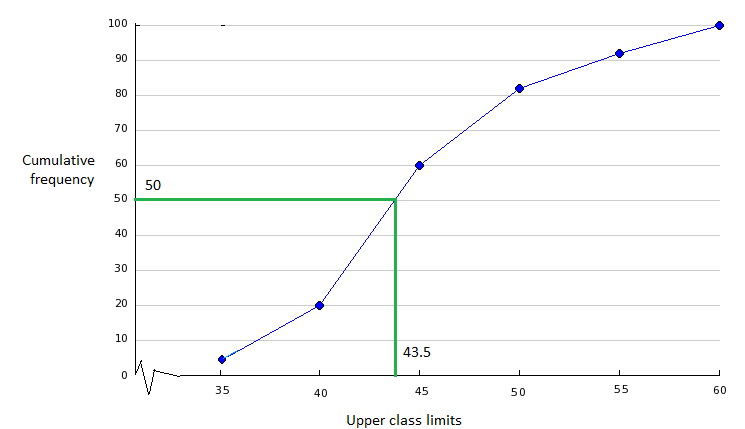Median = 43.5

Question 11

(a) A box contains 16 cards bearing numbers 1, 2, 3, …, 16, respectively. If a card is drawn at random from the box, find the probability that the number on the card is:

(i) an odd number

(ii) a prime number

(iii) a number not divisible by 4. 

Solution:

Given,

A box contains 16 cards bearing numbers 1, 2, 3, …, 16, respectively.

Sample space = S = {1, 2, 3, 4, …., 16}

n(S) = 16

(i) Let A be the event of getting an odd number.

A = {1, 3, 5, 7, 9, 11, 13, 15}

n(A) = 8

P(A) = n(A)/ n(S)

= 8/16

= 1/2

(ii) Let B be the event of getting a prime number.

B = {2, 3, 5, 7, 11, 13}

n(B) = 6

P(B) = n(B)/ n(S)

= 6/16

= 3/8

(iii) Let C be the event of getting a number not divisible by 4.

C = {1, 2, 3, 5, 6, 7, 9, 10, 11, 13, 14, 15}

n(C) = 12

P(C) = n(C)/ n(S)

= 12/16

= 3/4

(b) Prove that: cot A – tan A = (2 cos2A – 1)/ (sin A cos A). 

Solution:

LHS = cot A – tan A

= (cos A/ sin A) – (sin A/ cos A)

= (cos2A – sin2A)/ (sin A cos A)

Using the identity sin2θ + cos2θ = 1,

= [cos2A – (1 – cos2A)] / (sin A cos A)

= (cos2A – 1 + cos2A)/ (sin A cos A)

= (2 cos2A – 1)/ (sin A cos A)

= RHS

Hence proved.

(c) Find the mean of the following data:

 Class 0 – 10 10 – 20 20 – 30 30 – 40 40 – 50 Frequency 12 16 6 7 9


Solution:

 Class Frequency (f) Class mark (x) fx 0 – 10 12 5 60 10 – 20 16 15 240 20 – 30 6 25 150 30 – 40 7 35 245 40 – 50 9 45 405 ∑f = 50 ∑fx = 1100

Mean = ∑fx/ ∑f

= 1100/ 50

= 110/5

= 22

Therefore, the mean is 22.